• 机器学习的讲义，主要介绍利用矩阵和最大似然估计推导最小均方误差函数
• MSE均方误差，用来检测模型的预测值与真实值之间的偏差，值越大表示预测效果越差，可在程序中通过程序名直接调用。适用于初学者学习测试matlab MSE
• 本文将从代价函数的个人理解，均方误差代数函数作用原理以及在线性回归问题中为什么选择均方误差代数函数阐述。 1、代价函数的理解： 代价函数：真实的值与预测的值之间的偏差，由于偏差有可能正有可能负，因此使用...
在机器学习中，线性回归、逻辑回归问题总是绕不开代价函数。本文将从代价函数的个人理解，均方误差代数函数作用原理以及在线性回归问题中为什么选择均方误差代数函数阐述。
1、代价函数的理解：
代价函数：真实的值与预测的值之间的偏差，由于偏差有可能正有可能负，因此使用均方差来表示。代价函数的目的即用于找到最优解。
损失函数Loss Function：定义在单个样本上，算的是一个样本的误差。 代价函数Cost Function：定义在整个训练集上，算的是所有样本的误差，也就是损失函数的平均。 目标函数Object Function：定义为最终需要优化的函数。 Object Function = Cost Function（经验风险） + 正则化项（结构风险）
2、均方误差代数函数作用原理：
对于回归问题，代价函数常选择均方误差函数。下面阐述其作用原理： 对于假设函数：

h

θ

(

x

)

=

θ

0

+

θ

1

x

h_{\theta}(x)=\theta_{0}+\theta_{1} x

参数

θ

0

\theta_{0}

和

θ

1

\theta_{1}

两个参数的变化会导致假设函数的变化: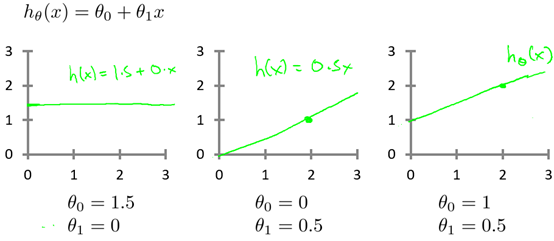实际的例子中，数据会以很多点的形式给出，回归问题就是将这些点拟合成一条曲线，即找到最优的参数

θ

0

\theta_{0}

和

θ

1

\theta_{1}

使得拟合的曲线更能代表所有的数据。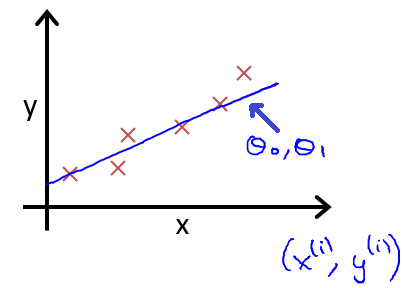如何找到最优参数

θ

0

\theta_{0}

和

θ

1

\theta_{1}

呢？使用代价函数！以均方误差代数函数为例： 从最简单的单一参数来看，假设函数为：

h

θ

(

x

)

=

θ

1

x

h_{\theta}(x)=\theta_{1} x

均方误差代数函数的主要思想就是将实际数据给出的值与拟合出的线的对应的值做差，求出拟合出的直线与实际的差距。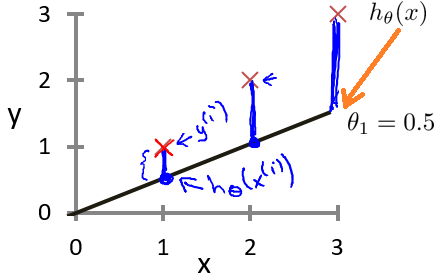为了使这个值不受个别极端数据的影响而产生巨大波动，采用类似方差再取二分之一的方式来减小个别数据影响。 这样，就产生了代价函数：

J

(

θ

0

,

θ

1

)

=

1

2

m

∑

i

=

1

m

(

h

θ

(

x

(

i

)

)

−

y

(

i

)

)

2

J\left(\theta_{0},\theta_{1}\right)=\frac{1}{2m}\sum_{i=1}^{m}\left(h_{\theta}\left(x^{(i)}\right)-y^{(i)}\right)^{2}

而最优解即为代价函数的最小值，根据以上公式多次计算可得到代价函数的图像：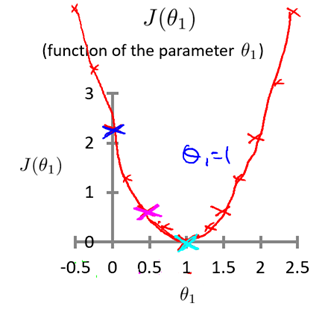可以轻易知道该代价函数的最小值(如何求解最小值？梯度下降算法~)，这时可以解出对应的 如果更多参数的话，图像就会更为复杂，两个参数的时候就已经是三维图像：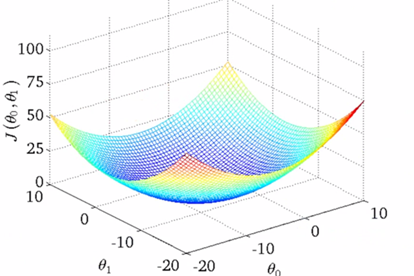高度即为均方误差代价函数的值，若参数再多则无法进行可视化，但其原理是相似的。 因此，我们可以终结得到：对于回归问题的代价函数，其最小值为：

minimize

⁡

θ

0

,

θ

1

J

(

θ

0

,

θ

1

)

\underset{\theta_{0}, \theta_{1}}{\operatorname{minimize}} J\left(\theta_{0}, \theta_{1}\right)

3、在回归问题中为什么选择均方误差函数：
从代价函数的理解考虑：代价是预测值和实际值之间的差距（两点之差），对于多个样本来说，就是差距之和。 将每一个样本的预测值和实际值之差相加，这不就是代价？但是注意到就每一个样本而言，代价有正有负，所有样本的代价加起来有可能出现正负抵消的情况，所以这并不是一个合适的代价函数（指将每一个样本的预测值和实际值之差相加）。 解决有正负的问题： 使用绝对值函数来表示代价，为了方便计算最小代价（计算最小代价可能用到最小二乘法），直接使用平方来衡量代价，即使用绝对值的平方来表示单个样本的代价，那么一个数据集的代价为：

(

h

θ

(

x

(

i

)

)

−

y

(

i

)

)

2

\left(h_{\theta}\left(x^{(i)}\right)-y^{(i)}\right)^{2}

即代价函数为：

J

(

θ

0

,

θ

1

)

=

1

2

m

∑

i

=

1

m

(

h

θ

(

x

(

i

)

)

−

y

(

i

)

)

2

J\left(\theta_{0},\theta_{1}\right)=\frac{1}{2m}\sum_{i=1}^{m}\left(h_{\theta}\left(x^{(i)}\right)-y^{(i)}\right)^{2}

为什么分母取2

m

m

而非

m

m

，是为了计算方便。
展开全文代价函数 线性回归
• mse是检验神经网络算法的误差分析。 代码举例： [x,t] = house_dataset; net = feedforwardnet(10); net.performFcn = 'mse'; % Redundant, MSE is default net.performParam.regularization = 0.01; net = train...
mse是检验神经网络算法的误差分析。 代码举例： [x,t] = house_dataset; net = feedforwardnet(10); net.performFcn = 'mse'; % Redundant, MSE is default net.performParam.regularization = 0.01; net = train(net,x,t); y = net(x); perf = perform(net,t,y); Alternately, you can call this function directly. perf = mse(net,x,t,'regularization',0.01); 对于简单的数值没有必要用这么复杂神经网络（主要是没法用，mse不是处理简单数据的函数） 对于你而言简单的用sum((Y-T).^2)就可以了
展开全文• m是训练的样本数量 i表示第i个样本 均方误差函数就是把预测值和真实值差的平方求和再除以2倍的样本数量 其中取2m而不取m是为了方便计算梯度. 用一道题帮助我们理解代价函数: 这道题给了三个样本点，并且给出假设函数...
均方误差代价函数 MSE Cost Function
代价函数可以用来衡量假设函数(hypothesis function)的准确性
在线性回归中，如果假设函数为h(x)=θ0+θ1*x, 均方误差代价函数公式如下图: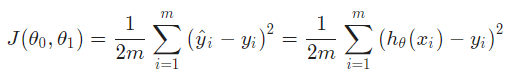θ0 和 θ1是假设函数的参数 h(x)就是预测值 m是训练的样本数量 i表示第i个样本
均方误差函数就是把预测值和真实值差的平方求和再除以2倍的样本数量
其中取2m而不取m是为了方便计算梯度.
用一道题帮助我们理解代价函数: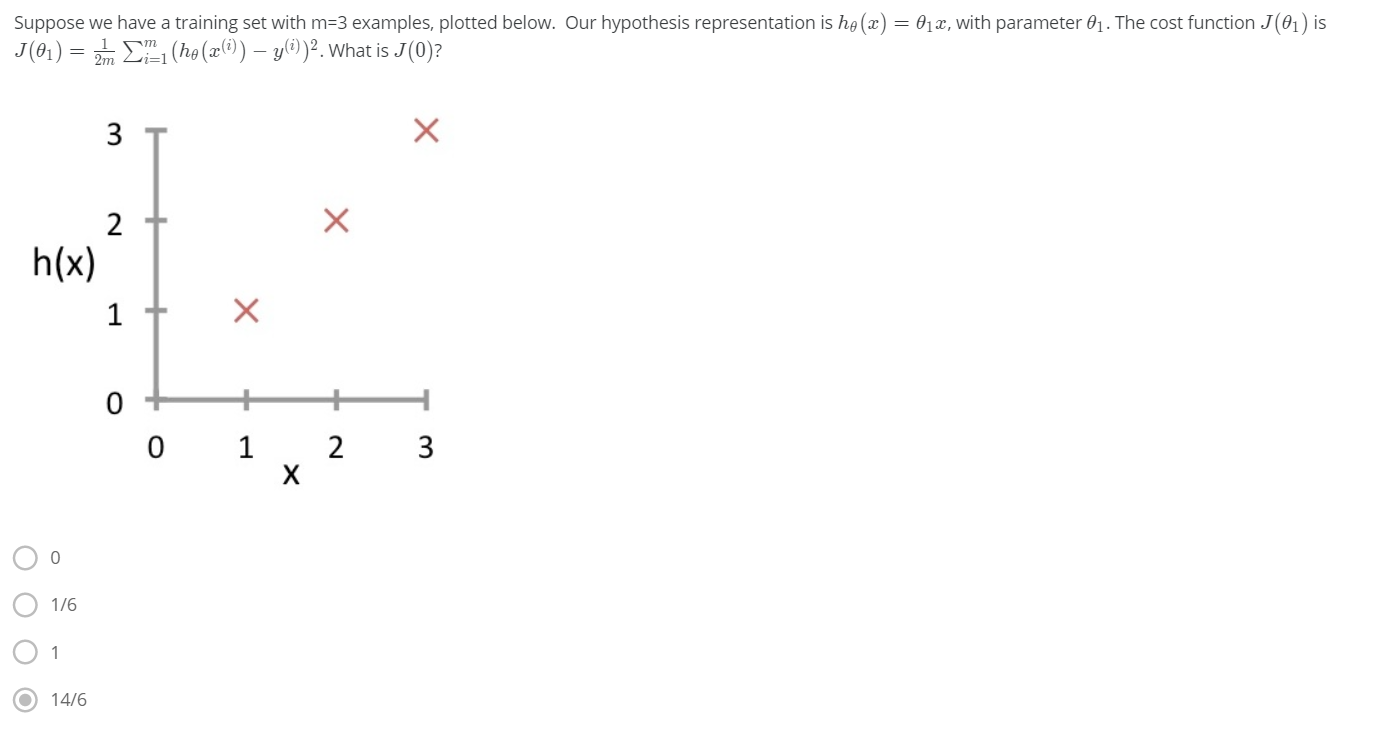这道题给了三个样本点，并且给出假设函数h(x)=θ1x,代价函数是均方误差代价函数，求J(0) 由题可知，θ0=0，要求J(0)，就是让θ1=0，当θ1=0的时候，其实h(x)=0 那么根据公式可以计算，选D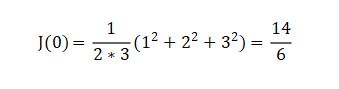当代价函数J(θ0，θ1)最小的时候，假设函数的预测值和真实值最接近 如下图，给定三个样本点(1,1),(2,2),(3,3),对于h(x)=0+θ1x,当θ1=1时，J(θ0,θ1)=0,即预测值和真实值完全相同，当θ1不为1时，预测的偏差随着θ1距离1的远近程度增加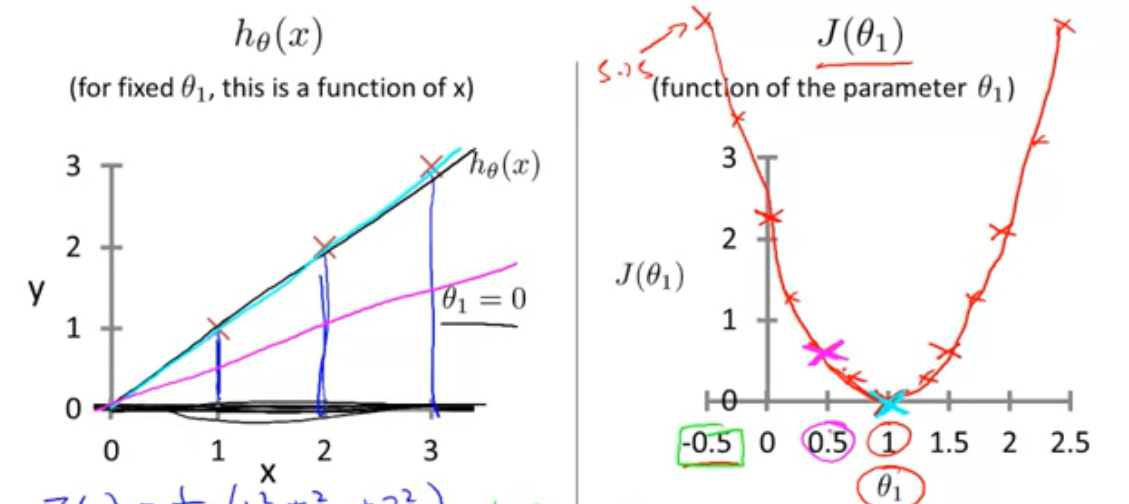展开全文机器学习
• 最小均方误差函数 第一讲里，我们提到了最小均方误差函数，给出一组有 mm 个样本的训练集，我们希望找到合适的参数 θ\boldsymbol{\theta}， 使得预测值 hθ(x)h_\theta(x) 与目标值尽可能接近。为了估计参数 θ\...
最小均方误差函数
之前的讲义里， 我们提到了最小均方误差函数，给出一组有

m
$m$ 个样本的训练集，我们希望找到合适的参数 θ$\boldsymbol{\theta}$， 使得预测值

hθ(x)
$h_\theta(x)$ 与目标值尽可能接近。为了估计参数

θ
$\boldsymbol{\theta}$， 我们定义如下的 cost function:

J(θ)=12∑i=1m(hθ(xi)−yi)2

\begin{equation}
J(\boldsymbol{\theta})=\frac{1}{2}\sum_{i=1}^{m}(h_\theta(\mathbf{x}_i)-y_i)^2
%\label{equ1}
\end{equation}
这个
cost function 就是最小均方误差函数，第一讲里面，我们用梯度下降算法估计参数

θ
$\boldsymbol{\theta}$, 而第二讲里面我们提到了矩阵的基本运算及矩阵的求导，现在就从矩阵的角度求参数

θ
$\boldsymbol{\theta}$的解析解。

矩阵的解析解
给定一组训练样本，

D={(xi,yi)|xi∈Rn,yi∈R}mi=1
$\mathbf{D}=\{(\mathbf{x}_{i},y_{i})| \mathbf{x}_{i}\in \mathfrak{R}^{n}, y_{i}\in \mathfrak{R}\}_{i=1}^{m}$,

xi
$\mathbf{x}_{i}$ 是维数为

n
$n$ 的输入特征(默认为列向量)，yi$y_{i}$ 是连续的实数值，

m
$m$表示样本数，则输入特征可以用矩阵表示为：
X=[x1,x2,⋯,xm]T\begin{equation*}
\mathbf{X}={\begin{bmatrix}
\mathbf{x}_{1}, & \mathbf{x}_{2},  & \cdots , & \mathbf{x}_{m}
\end{bmatrix}}^{T}
\end{equation*}  输出变量可以用一个向量表示为：

y=[y1,y2,⋯,ym]T

\begin{equation*}
\mathbf{y}={\begin{bmatrix}
{y}_{1}, & {y}_{2},  & \cdots , & {y}_{m}
\end{bmatrix}}^{T}
\end{equation*}
而我们从第一讲已经知道，

hθ(xi)=xTiθ
$h_\theta(\mathbf{x}_i)=\mathbf{x}_{i}^{T} \boldsymbol{\theta}$, 则我们可以得到如下的表达式：

Xθ−y=⎡⎣⎢⎢xT1θ⋮xTmθ⎤⎦⎥⎥−⎡⎣⎢⎢y1⋮ym⎤⎦⎥⎥=⎡⎣⎢⎢hθ(x1)−y1⋮hθ(xm)−ym⎤⎦⎥⎥

\begin{equation*}
\mathbf{X}\boldsymbol{\theta}-\mathbf{y} = \begin{bmatrix}
\mathbf{x}_{1}^{T} \boldsymbol{\theta} \\
\vdots \\
\mathbf{x}_{m}^{T} \boldsymbol{\theta} \\
\end{bmatrix}  - {\begin{bmatrix}
{y}_{1} \\
\vdots \\
{y}_{m} \\
\end{bmatrix}} = {\begin{bmatrix}
h_\theta(\mathbf{x}_1)-{y}_{1} \\
\vdots \\
h_\theta(\mathbf{x}_m)-{y}_{m} \\
\end{bmatrix}}
\end{equation*}
则最小均方误差函数可以表示为：

J(θ)=12∑i=1m(hθ(xi)−yi)2=12(Xθ−y)T(Xθ−y)

\begin{equation*}
J(\boldsymbol{\theta})=\frac{1}{2}\sum_{i=1}^{m}(h_\theta(\mathbf{x}_i)-y_i)^2=\frac{1}{2} (\mathbf{X}\boldsymbol{\theta}-\mathbf{y})^{T} (\mathbf{X}\boldsymbol{\theta}-\mathbf{y})
\end{equation*}
利用第二讲的矩阵基本运算将上式展开，可以得到：

J(θ)=12θTXTXθ−θTXTy−yTXθ+yTy

\begin{equation*}
J(\boldsymbol{\theta})=\frac{1}{2} \boldsymbol{\theta}^{T}\mathbf{X}^{T}\mathbf{X}\boldsymbol{\theta}-\boldsymbol{\theta}^{T}\mathbf{X}^{T} \mathbf{y}
-\mathbf{y}^{T}\mathbf{X}\boldsymbol{\theta}+\mathbf{y}^{T} \mathbf{y}
\end{equation*}
利用第二讲的矩阵求导，可以得到

J(θ)
$J(\boldsymbol{\theta})$ 对

θ
$\boldsymbol{\theta}$ 的偏导数为：

∂J(θ)∂θ=12(XTXθ+XTXθ−2XTy)=XTXθ−XTy

\begin{equation*}
\frac{\partial J(\boldsymbol{\theta})}{\partial \boldsymbol{\theta}}=\frac{1}{2} (\mathbf{X}^{T}\mathbf{X}\boldsymbol{\theta} + \mathbf{X}^{T}\mathbf{X}\boldsymbol{\theta} -2 \mathbf{X}^{T} \mathbf{y})=\mathbf{X}^{T}\mathbf{X}\boldsymbol{\theta}-\mathbf{X}^{T} \mathbf{y}
\end{equation*}
令该偏导数为0，则可以得到：

XTXθ=XTy

\begin{equation*}
\mathbf{X}^{T}\mathbf{X}\boldsymbol{\theta}=\mathbf{X}^{T} \mathbf{y}
\end{equation*}
最终可以得到参数

θ
$\boldsymbol{\theta}$ 的解析解为：

θ=(XTX)−1XTy

\begin{equation*}
\boldsymbol{\theta}=(\mathbf{X}^{T}\mathbf{X})^{-1}\mathbf{X}^{T} \mathbf{y}
\end{equation*}
如果矩阵

(XTX)−1
$(\mathbf{X}^{T}\mathbf{X})^{-1}$ 可逆，则该解就是全局最优解，有的时候，该矩阵不一定可逆，就是我们常常遇到的样本数远远大于参数的个数即

m≫n
$m\gg n$,那么参数

θ
$\boldsymbol{\theta}$ 就只能得到近似解。

从最大似然估计到最小均方误差
有的时候我们会考虑，在做回归问题的时候，为什么用最小均方误差函数作为 \textbf{cost function}, 为什么不用其它的函数。接下来，我们从概率分布的角度去考虑这个问题，随后我们会发现，最小均方误差函数从某种意义上来说，  是从最大似然估计中演化出来的。  我们先假设目标值与输入变量之间存在以下关系：

yi=θTxi+ϵi

\begin{equation}
y_{i}=\boldsymbol{\theta}^{T} \mathbf{x}_{i} + \epsilon_{i}
\end{equation}
其中，

ϵi
$\epsilon_{i}$是一个误差项，表示由于一些我们没有考虑到的因素导致估计值域目标值之间出现得偏差，我们进一步假设这些误差项是
独立同分布的(independently and identically distributed), 并且是均值为0，方差为

σ2
$\sigma^{2}$ 的高斯分布(这是自然界非常常见的一种分布)。我们可以表示为

ϵi∼N(0,σ2)
$\epsilon_{i} \sim N(0,\sigma^{2})$, 其概率密度函数为：

p(ϵi)=12π−−√σexp(−ϵ2i2σ2)

\begin{equation*}
p(\epsilon_{i})=\frac{1}{\sqrt{2 \pi}\sigma}exp \left(- \frac{\epsilon_{i}^{2}}{2\sigma^{2}} \right)
\end{equation*}
将式代入，可以得到：

p(yi|xi;θ)=12π−−√σexp(−(yi−θTxi)22σ2)

\begin{equation*}
p(y_{i}|\mathbf{x}_{i}; \boldsymbol{\theta})=\frac{1}{\sqrt{2 \pi}\sigma}exp(- \frac{(y_{i}-\boldsymbol{\theta}^{T} \mathbf{x}_{i})^{2}}{2\sigma^{2}})
\end{equation*}
这里需要注意，

p(yi|xi;θ)
$p(y_{i}|x_{i}; \boldsymbol{\theta})$表示的是

yi
$y_{i}$对

xi
$\mathbf{x}_{i}$的条件概率，

θ
$\boldsymbol{\theta}$是参数，

xi
$\mathbf{x}_{i}$是随机变量，两者之间用

";"
$";"$隔开，不能用

","
$","$隔开。当我们考虑所有的样本时，则所有的输出变量的分布表示为：

p(y|X;θ)
$p(\mathbf{y}|\mathbf{X}; \boldsymbol{\theta})$, 我们可以建立一个关于参数

θ
$\boldsymbol{\theta}$的似然函数：

L(θ)=L(θ;X,y)=p(y|X;θ)

\begin{equation*}
L(\boldsymbol{\theta})=L(\boldsymbol{\theta};\mathbf{X}, \mathbf{y})=p(\mathbf{y}| \mathbf{X}; \boldsymbol{\theta})
\end{equation*}
因为

ϵi
$\epsilon_{i}$ 是独立同分布的，我们可以得到：

L(θ)=∏i=1mp(yi|xi;θ)=∏i=1m12π−−√σexp(−(yi−θTxi)22σ2)

\begin{equation*}
L(\boldsymbol{\theta})=\prod_{i=1}^{m}p(y_{i}|\mathbf{x}_{i}; \boldsymbol{\theta})=\prod_{i=1}^{m}\frac{1}{\sqrt{2 \pi}\sigma}exp \left( - \frac{(y_{i}-\boldsymbol{\theta}^{T} \mathbf{x}_{i})^{2}}{2\sigma^{2}} \right)
\end{equation*}
最大似然估计原则告诉我们，要选择参数

θ
$\boldsymbol{\theta}$使得概率要尽可能地高。所以我们可以建立如下的优化函数：

maxθ[∏i=1m12π−−√σexp(−(yi−θTxi)22σ2)]

\begin{equation}
\max_{\boldsymbol{\theta}} \left[ \prod_{i=1}^{m}\frac{1}{\sqrt{2 \pi}\sigma}exp \left( - \frac{(y_{i}-\boldsymbol{\theta}^{T} \mathbf{x}_{i})^{2}}{2\sigma^{2}} \right) \right ]
\end{equation}
上式是一个单调函数，根据单调函数的传递性原则，我们可以对上式取对数，则可以得到：

l(θ)=log(L(θ))=log∏i=1m12π−−√σexp(−(yi−θTxi)22σ2)=∑i=1mlog12π−−√σexp(−(yi−θTxi)22σ2)=mlog12π−−√σ−1σ2⋅12∑i=1m(yi−θTxi)2

\begin{equation}
\begin{split}
\mathfrak {l}(\boldsymbol{\theta}) =log(L(\boldsymbol{\theta}))
& = \text{log} \prod_{i=1}^{m}\frac{1}{\sqrt{2 \pi}\sigma}exp \left( - \frac{(y_{i}-\boldsymbol{\theta}^{T} \mathbf{x}_{i})^{2}}{2\sigma^{2}} \right)  \\
& = \sum_{i=1}^{m} \text{log} \frac{1}{\sqrt{2 \pi}\sigma}exp \left( - \frac{(y_{i}-\boldsymbol{\theta}^{T} \mathbf{x}_{i})^{2}}{2\sigma^{2}} \right) \\
& = m \text{log} \frac{1}{\sqrt{2 \pi}\sigma}- \frac{1}{\sigma^{2}} \cdot \frac{1}{2} \sum_{i=1}^{m} (y_{i}-\boldsymbol{\theta}^{T} \mathbf{x}_{i})^{2}
\end{split}
\end{equation}
因此，要使上式最大化，则只要使其中的二次项最小化，即

minθ12∑i=1m(hθ(xi)−yi)2

\begin{equation*}
\min_{\boldsymbol{\theta}}\frac{1}{2}\sum_{i=1}^{m}(h_\theta(\mathbf{x}_i)-y_i)^2
\end{equation*}
我们可以看到，从概率出发，最小均方误差是最大似然估计非常自然的一种延伸，两者最后可以完美的统一起来。

参考文献

Andrew Ng, “Machine Learning”, Stanford University.

展开全文机器学习
• mse(a) ----------------------------函数...MSE是（神经）网络的均方误差，叫"Mean Square Error"。比如有n对输入输出数据，每对为[Pi,Ti],i=1,2,...,n.网络通过训练后有网络输出,记为Yi。那MSE＝(求和(Ti-Yi)^2(i=1
• 一、均方误差损失函数 对于回归问题，最常用的损失函数就是均方误差损失函数（MSE） 定义如下： 其中yi为输出中第i个数据的答案值，yi‘ 就是神经网络给出的预测值。因此最小化该函数就是优化的目标。 通过最小...深度学习 tensorflow 机器学习 python
• tensorflow均方误差损失函数 引用API：tensorflow.kreas.losses.MSE 均方误差（Mean Square Erroe）是回归问题最常用的损失函数，回归问题解决的是对具体数值的预测，而不是预测某个事先定义好的分类 则，...机器学习 tensorflow 深度学习 python 神经网络
• 计算均方误差MSE信噪比SNR峰值信噪比PSNR绝对平均误差的matlab函数
• 均方误差的公式是E=½∑(yk-tk)²,yk代表神经网络的输出,tk代表监督数据,k表示数据的维数,神经网络的输出含义是经过了某种运算得出的最终结果,监督数据指的是正确的数据用来与最终输出相比较,维数的含义则是,得出的...python 人工智能 神经网络 数学
• 误差函数理解定义功能与BP算法，激活函数的关系误差函数的特点常见误差函数均方误差函数公式应用场景pytorch实现代码交叉熵公式应用场景pytorch实现代码 定义 误差函数是关于模型输出和样本标签值之差的函数，通过对...
• 交叉熵（cross entropy）是常用的方法之一，刻画了两个概率分布之间的距离，是分类问题中使用较多的一种损失函数。 给定两个概率分布p和q，通过q来表示p的交叉熵为： 如何将神经网络前向传播得到的结果也变成...神经网络 MSE
• ## Matlab 计算均方误差MSE的三种方法

万次阅读 多人点赞 2020-11-02 23:26:34
Matlab 计算均方误差MSE的三种方法 数据说明： ytest 测试集y，真实的y值，是一维数组； ytest_fit 基于测试集 x 预测的y值，是一维数组； test_error 是预测误差。 第一种方法 直接使用 matlab 中的mse函数，亲测...matlab mse
• torch.nn.MSELoss 类使用均方误差函数对损失值进行计算，在定义类的对象时不用传入任何参数，但在使 用实例时需要输入两个维度一样的参数方可进行计算。示例如下： import torch as t loss_f = t.nn.MSELoss() x =...
• :https://www.jianshu.com/p/c7e642877b0ePython实现
• 首先从可行性的角度想到的是均方误差函数是光滑函数，能够采用梯度下降算法进行优化。但这似乎不是一个好的解释。而从概率统计的角度来理解，其背后的最终支撑是最小二乘估计假设误差是服从高斯分布的，为什么这...
• 我们设计神经网络的目的是为了实现一种模型，这个模型能够对数据做出合适的处理。比如分类问题，网络能够 以尽可能高的准确...首先，先说一下MSE，均方误差函数，这个函数在很多地方（常见于线性回归问题上，针对不...神经网络 算法
• 使用matlab对图象计算均方误差使用matlab对图象计算均方误差matlab
• MSE（均方误差函数一般用来检测模型的预测值和真实值之间的偏差。 训练集：Train=｛（x1，y1），（x2，y2），...,(xn,yn),...,(xN,yN)} N为训练样本总数，n=1，2，...,N。 测试集：Test=｛（x1，y1），（x2，y....
• 与分类问题不同，回归问题解决的是对具体数值的预测，eg：房价预测，销量预测，流量预测等均方误差的定义：均方误差#y代表输出答案，y_代表标准答案 mse=tf.reduce_mean(tf.square(y_-y))但在实际问题中往往均方误差...
• 比如对于一个4分类问题，期望输出应该为g0=[0,1,0,0]，实际输出为g1=[0.2,0.4,0.4,0]，计算g1与g0之间的差异所使用的方法，就是损失函数，分类问题中常用损失函数是交叉熵。 交叉熵（cross entropy）描述的是两个...
• 在经过以上 激活函数后，线性回归选用均方误差为损失函数，逻辑回归选择交叉熵为损失函数。以下代码使用tensorflow实现。 softmax激活函数：用在多分类，一个输入只能有一个输出，输出标签独立（onehot类型标签，...loss
• MSE均方误差（L2 loss） 1.代码展示MAE和MSE图片特性 import tensorflow as tf import matplotlib.pyplot as plt sess = tf.Session() x_val = tf.linspace(-1.,-1.,500) target = tf.constant(0....
• MSE（均方误差）对于每一个输出的结果都非常看重，而交叉熵只对正确分类的结果看重。 例如：在一个三分类模型中，模型的输出结果为（a,b,c)，而真实的输出结果为(1,0,0)，那么MSE与cross-entropy相对应的损失函数的......